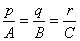§4  空间中的直线与平面

 名  称  与  说  明 图  形 [方向角]        通过原点O的直线OM与三条坐标轴的夹角a, b, g 称为该直线的方向角(OM的方向为离开原点O的方向)：a =∠MOx，b =∠MOy，g =∠MOz [方向余弦]        直线的方向角的余弦称为方向余弦：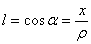，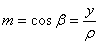，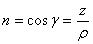式中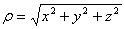，l2 + m2 + n2 = 1[方向数]        通过原点且平行于直线L的直线OM上任意一点W的坐标(p, q, r)称为直线L的方向数，而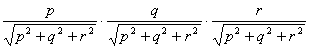为直线OM的方向余弦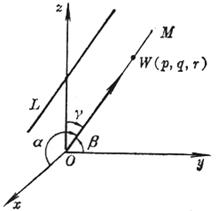名  称  与  说  明 图  形 [过两点的直线的方向余弦]，，式中这时直线的正向为M1(x1, y1, z1)到M2(x2, y2, z2)的方向.方  程  与  图  形 说  明 [截距式]a, b, c分别称为平面在三条坐标轴上的截距 [点法式](A, B, C不同时等于零)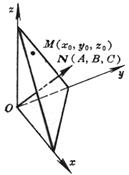平面通过点M(x0, y0, z0)，且法线N的方向数为A, B, C [三点式]平面通过三点:          M1(x1, y1, z1)          M2(x2, y2, z2)          M3(x3, y3, z3) 或=0 方  程 与  图  形 说  明 [一般式]   Ax + By + Cz + D = 0   (A, B, C为该平面的法线的方向数，且不同时等于零)当D=0时，平面通过原点        当A=0时(或B=0，或C=0)时，平面与x轴(或y轴，或z轴)平行        当A=B=0时(或A=C=0，或B=C=0)时，平面与Oxy平面(或Ozx，或Oyz)平行 [法线式]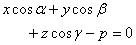(a, b, g为平面的法线的方向角，p³0为法线长即原点到平面的距离)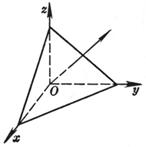平面的一般式可化为法线式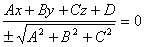，式中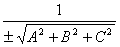称为平面的法化因子，当D< 0时取正号；D>0时取负号 [矢量式] (r -r0)× a = 0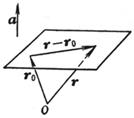平面 通过矢径r0的终点，且与已知矢量a垂直，r为平面上任意一点的矢径

 方  程  与  图  形 说  明 [一般式(或交面式)] L把直线L作为两个平面的交线，它的方向数为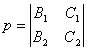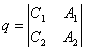[对称式(或参数式)]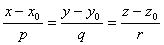或直线L通过点M(x0, y0, z0)，且具有方向数p, q, r 方  程  与  图  形 说  明 [两点式]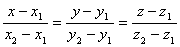直线L通过M1(x1, y1, z1)和M2(x2, y2, z2)两点 [射影式] L直线L是y = ax+ g 和z = bx + h两个平面的交线；通过点(0, g, h)且具有方向数1, a, b [矢量式]   r = r0 + ta   (-¥< t < ¥)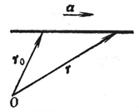直线L通过矢径r0的终点，且与已知矢量a平行，r为L上任意一点的矢径

 方  程  与  图  形 公  式  与  说  明 [二平面的夹角]  P1 A1x + B1y + C1z + D1 = 0  P2 A2x + B2y + C2z + D2 = 0式中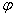就是二平面P1和P2的二面角 方  程  与  图  形 公  式  与  说  明 [平面束×三平面共线的条件] Pl   (A1x+ B1y + C1z + D1) + l  (A2x + B2y + C2z + D2) = 0   (l为参数，-¥

[直线与平面的平行与垂直条件]

 平  行  条  件 垂  直  条  件 线与线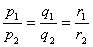面与面线与面p1p2 + q1q2 + r1r2 = 0          A1A2 + B1B2 + C1C2 = 0